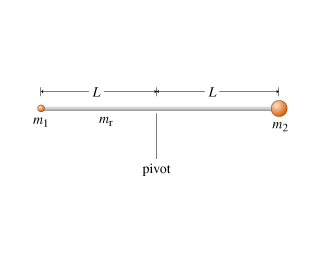# Problem: A thin rod of mass mr and length 2L is allowed to pivot freely about its center, as shown in the diagram. A small sphere of mass m1 is attached to the left end of the rod, and a small sphere of mass m2 is attached to the right end. The spheres are small enough that they can be considered point particles. The gravitational force acts downward, with the magnitude of the gravitational acceleration equal to g.Part AWhat is the moment of inertia I of this assembly about the axis through which it is pivoted?Express the moment of inertia in terms of mr, m1, m2, and L. Remember, the length of the rod is 2L, not L.Part BSuppose the rod is held at rest horizontally and then released. (Throughout the remainder of this problem, your answer may include the symbol I, the moment of inertia of the assembly, whether or not you have answered the first part correctly.)What is the angular acceleration α of the rod immediately after it is released?Take the counterclockwise direction to be positive. Express α in terms of some or all of the variables mr, m1, m2, L, I, and g.

###### FREE Expert Solution

Part A

Moment of inertia of a thin rod about its center is:

$\overline{){\mathbf{I}}{\mathbf{=}}\frac{{\mathbf{mL}}^{\mathbf{2}}}{\mathbf{12}}}$

Therefore,

79% (171 ratings)###### Problem Details

A thin rod of mass mr and length 2L is allowed to pivot freely about its center, as shown in the diagram. A small sphere of mass m1 is attached to the left end of the rod, and a small sphere of mass m2 is attached to the right end. The spheres are small enough that they can be considered point particles. The gravitational force acts downward, with the magnitude of the gravitational acceleration equal to g.Part A

What is the moment of inertia I of this assembly about the axis through which it is pivoted?

Express the moment of inertia in terms of mr, m1, m2, and L. Remember, the length of the rod is 2L, not L.

Part B

Suppose the rod is held at rest horizontally and then released. (Throughout the remainder of this problem, your answer may include the symbol I, the moment of inertia of the assembly, whether or not you have answered the first part correctly.)

What is the angular acceleration α of the rod immediately after it is released?

Take the counterclockwise direction to be positive. Express α in terms of some or all of the variables mr, m1, m2, L, I, and g.